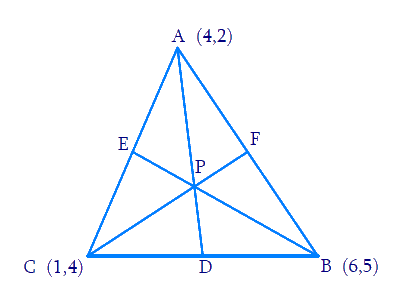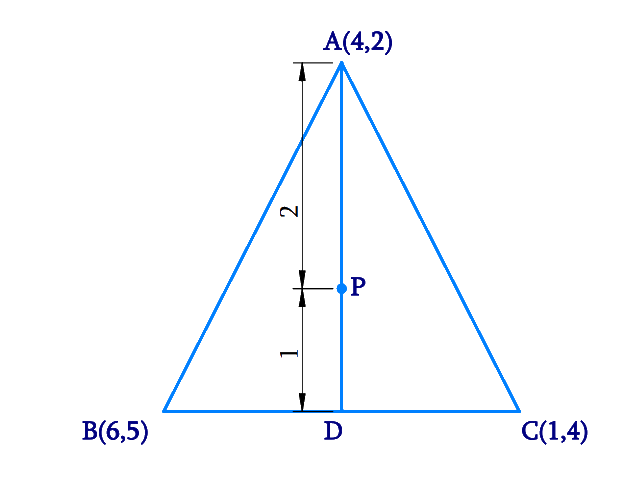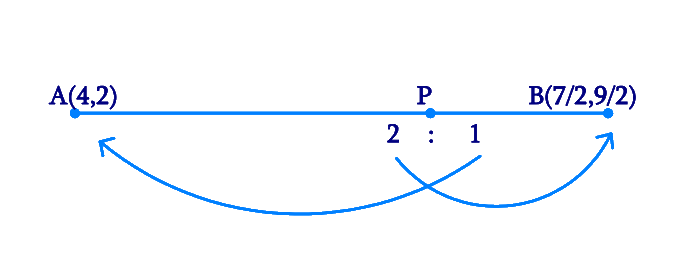# Ex.7.4 Q7 Coordinate Geometry Solution - NCERT Maths Class 10

Go back to  'Ex.7.4'

## Question

Let $$A\;(4, 2)$$, $$B \;(6, 5)$$ and $$C \;(1, 4)$$ be the vertices of $$\Delta ABC$$.

(i) The median from $$A$$ meets $$BC$$ at $$D$$. Find the coordinates of point $$D$$.

(ii) Find the coordinates of the point $$P$$ on $$AD$$ such that $$AP: PD = 2:1$$

(iii) Find the coordinates of point $$Q$$ and $$R$$ on medians $$BE$$ and $$CF$$ respectively such that $$BQ: QE = 2:1$$ and $$CR: RF = 2:1$$.

(iv) What do you observe?

(v) If $$A(x_1, y_1)$$, $$B(x_2, y_2)$$, and $$C(x_3, y_3)$$ are the vertices of $$\Delta ABC$$, find the coordinates of the centroid of the triangle.

Video Solution
Coordinate Geometry
Ex 7.4 | Question 7

## Text Solution

Reasoning:

A median of a triangle is a line segment joining the vertex to the midpoint of the opposite side, thus bisecting that side. Every triangle has exactly three medians, one from each vertex, and they all intersect each other at the triangle's centroid.

What is known?

The $$x$$ and $$y$$ coordinates of the vertices of the triangle.

What is unknown?

The coordinates of point $$D$$, $$P$$, $$Q$$, $$R$$ and the co-ordinates of the centroid.

Steps:

From the figure,Given,

• Let \begin{align}A \left( {{x_1}, {y_1}} \right) = \left( {4, 2} \right)\end{align}
• Let \begin{align}B \left( {{x_2}, {y_2}} \right) = \left( {6 , 5} \right)\end{align}
• Let \begin{align}C\left( {{x_3}, {y_3}} \right) = \left( {1, 4} \right)\end{align}

(i)

• Let $$AD$$ be the median of the triangle $$ABC$$
• Hence $$D$$ is the midpoint of $$BC$$ which can be found using the Mid Point formula.

\begin{align}M& = \left[ {\frac{{{x_1} + {x_2}}}{2},\frac{{{y_1} + {y_2}}}{2}} \right]\end{align}

Coordinates of $$D$$

\begin{align} &= \left[ {\frac{{6 + 1}}{2},\frac{{5 + 4}}{2}} \right] \\ &= \left[ {\frac{7}{2},\frac{9}{2}} \right]\end{align}

(ii) From the Figure,Point $$P$$ divides the side $$AD$$ in a ratio $$m: n = 2: 1$$.

By Section Formula

\begin{align} P(x,y) & \!\!= \!\!\left[\!\! {\frac{{m{x_2} \!+\! n{x_1}}}{{m \!+\! n}},\frac{{m{y_2} \!+\! n{y_1}}}{{m \!+\! n}}} \!\! \right] \dots(1)\end{align}

Coordinates of $$P$$

\begin{align} & \!\!=\!\! \left[ {\frac{{2 \times \frac{7}{2} + 1 \times 4}}{{2 + 1}},\frac{{2 \times \frac{9}{2} + 1 \times 2}}{{2 + 1}}} \right] \\ &= \left[ {\frac{{11}}{3},\frac{{11}}{3}} \right]\end{align}

(iii) From the figure,Median $$BE$$ of the triangle will divide the side $$AC$$ in two equal parts.

Therefore, $$E$$ is the mid-point of side $$AC$$. The co-ordinates of $$E$$ can be calculated using the Mid-Point Formula.

\begin{align}M &= \left[ {\frac{{{x_1} + {x_2}}}{2},\frac{{{y_1} + {y_2}}}{2}} \right] \end{align}

Coordinates of $$E$$

\begin{align} &= \left[ {\frac{{4 + 1}}{2},\frac{{2 + 4}}{2}} \right] \\ &= \left[ {\frac{5}{2},3} \right]\end{align}

Point $$Q$$ divides the side $$BE$$ in a ratio $$2:1$$.

By Section Formula

\begin{align} P(x,y) & \!\!= \!\!\left[ {\frac{{m{x_2} \!+\! n{x_1}}}{{m \!+\! n}}\!,\!\frac{{m{y_2} \!+\! n{y_1}}}{{m \!+\! n}}} \right] \! \dots \!(1) \end{align}

Coordinates of $$Q$$

\begin{align} & \!\!= \!\!\left[ {\frac{{2 \times \frac{5}{2} + 1 \times 6}}{{2 + 1}},\frac{{2 \times 3 + 1 \times 5}}{{2 + 1}}} \right] \\ & = \left[ {\frac{{11}}{3},\frac{{11}}{3}} \right]\end{align}

Median $$CF$$ of the triangle will divide the side $$AB$$ in two equal parts.

Therefore, $$F$$ is the mid-point of side $$AB$$. The co-ordinates of $$F$$ can be calculated using the Mid-Point Formula.

Coordinates of $$F$$

\begin{align} &= \left[ {\frac{{4 + 6}}{2},\frac{{2 + 5}}{2}} \right] \\&= \left[ {5,\frac{7}{2}} \right]\end{align}

Point $$R$$ divides the side $$CF$$ in a ratio $$2: 1$$

By Section Formula

\begin{align} P(x,y) & \!\!= \!\!\left[ {\frac{{m{x_2}\! +\! n{x_1}}}{{m\! +\! n}}\!,\!\frac{{m{y_2} \!+\! n{y_1}}}{{m \!+\! n}}} \right] \!\dots \!(1) \end{align}

Coordinates of R

\begin{align} & \!\!= \!\!\left[ {\frac{{2 \times 5 + 1 \times 1}}{{2 + 1}},\frac{{2 \times \frac{7}{2} + 1 \times 4}}{{2 + 1}}} \right] \\ &= \left[ {\frac{{11}}{3},\frac{{11}}{3}} \right]\end{align}

(iv) It can be observed that the coordinates of point P, Q, R are the same. Therefore, all these are representing the same point on the plane i.e., the centroid of the triangle.

(v) Consider a triangle, $$\Delta ABC$$, having its vertices as

\begin{align}& A\left( {{x_1},\,{y_1}} \right),{\text{ }}B\left( {{x_2},\,{y_2}} \right),\end{align} and \begin{align}C\,\left( {{x_3},{y_3}} \right)\end{align}

Median $$AD$$ of the triangle will divide the side $$BC$$ in two equal parts.

Therefore, $$D$$ is the mid-point of side $$BC$$. The co-ordinates of $$D$$ can be calculated using the Mid-Point Formula.

Coordinates of  $$D$$

\begin{align} \!=\! \left[ {\frac{{{{x}_2} \!+\! {x_3}}}{2},\frac{{{{y}_2} \!+\! {y_3}}}{2}} \right]\end{align}

Let the centroid of this triangle be $$O$$.

Point $$O$$ divides the side $$AD$$ in a ratio $$2:1$$.

Coordinates of $$O$$

\begin{align} &\!=\! \left[ \frac{{2 \!\times\! \frac{{{x_2} +\! {x_3}}}{2} \!+\! 1 \!\times\! {x_1}}}{{2 \!+\! 1}}, \frac{{2 \!\times\! \frac{{{y_2} +\! {y_3}}}{2} \!+\! 1 \!\times\! {y_1}}}{{2 \!+\! 1}} \right] \\ &\!=\! \left[ {\frac{{{x_1} \!+\! {x_2} \!+\! {x_3}}}{3},\frac{{{y_1} \!+\! {y_2} \!+\! {y_3}}}{3}} \right]\end{align}

Learn from the best math teachers and top your exams

• Live one on one classroom and doubt clearing
• Practice worksheets in and after class for conceptual clarity
• Personalized curriculum to keep up with school